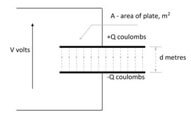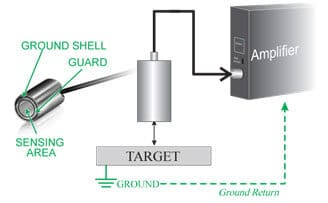## Capacitive displacement sensors

Capacitance works on the two plate parallel capacitance theory.Figure 3

Where the distance between plates d= ε*A/C

ε= dielectric constant of air, A = area of the plates, C = the capacitance (charge) between the plates.Figure 4